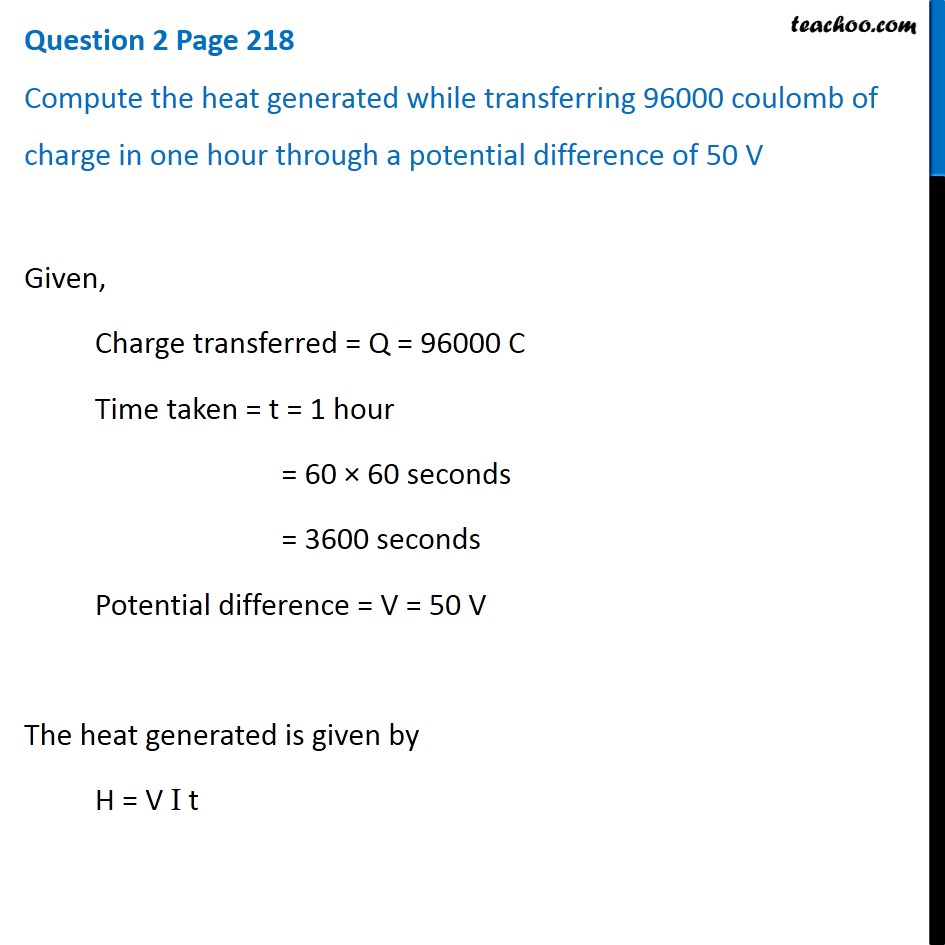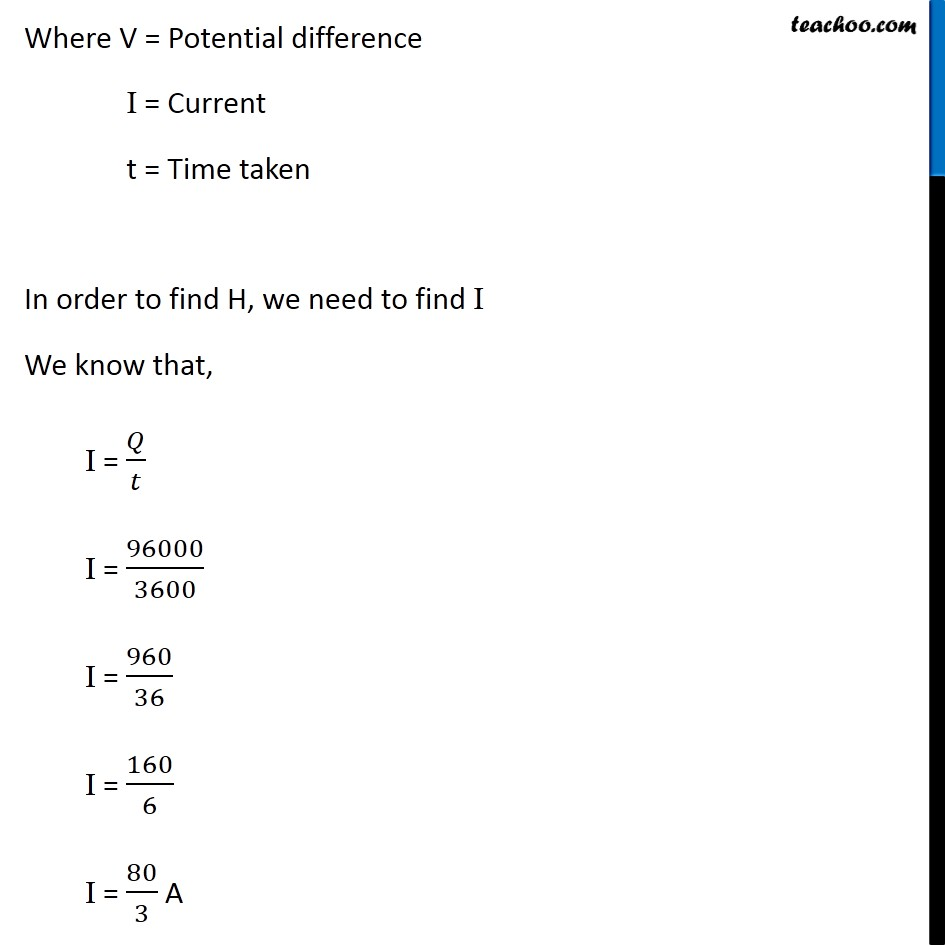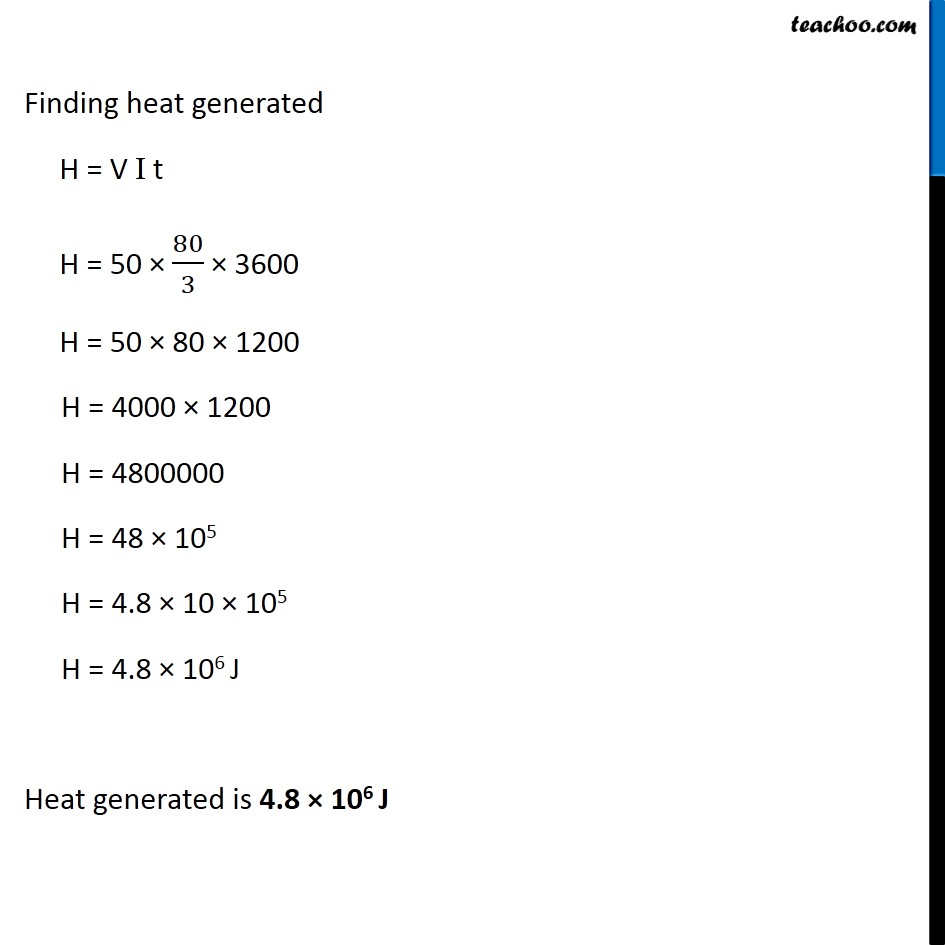Questions from Inside the chapter

Class 10
Chapter 12 Class 10 - Electricity

## Compute the heat generated while transferring 96000 coulomb of charge in one hour through a potential difference of 50 VLearn in your speed, with individual attention - Teachoo Maths 1-on-1 Class

### Transcript

Question 2 Page 218 Compute the heat generated while transferring 96000 coulomb of charge in one hour through a potential difference of 50 V Given, Charge transferred = Q = 96000 C Time taken = t = 1 hour = 60 × 60 seconds = 3600 seconds Potential difference = V = 50 V The heat generated is given by H = V I t Where V = Potential difference I = Current t = Time taken In order to find H, we need to find I We know that, I = 𝑄/𝑡 I = 96000/3600 I = 960/36 I = 160/6 I = 80/3 A Finding heat generated H = V I t H = 50 × 80/3 × 3600 H = 50 × 80 × 1200 H = 4000 × 1200 H = 4800000 H = 48 × 105 H = 4.8 × 10 × 105 H = 4.8 × 106 J Heat generated is 4.8 × 106 J